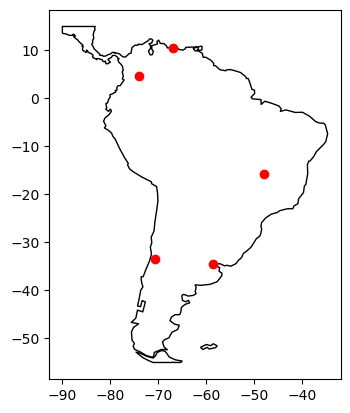# Creating a GeoDataFrame from a DataFrame with coordinates#

This example shows how to create a GeoDataFrame when starting from a regular DataFrame that has coordinates either WKT (well-known text) format, or in two columns.

:

import pandas as pd
import geopandas
import matplotlib.pyplot as plt
from geodatasets import get_path


# From longitudes and latitudes#

First, let’s consider a DataFrame containing cities and their respective longitudes and latitudes.

:

df = pd.DataFrame(
{
"City": ["Buenos Aires", "Brasilia", "Santiago", "Bogota", "Caracas"],
"Country": ["Argentina", "Brazil", "Chile", "Colombia", "Venezuela"],
"Latitude": [-34.58, -15.78, -33.45, 4.60, 10.48],
"Longitude": [-58.66, -47.91, -70.66, -74.08, -66.86],
}
)


A GeoDataFrame needs a shapely object. We use geopandas points_from_xy() to transform Longitude and Latitude into a list of shapely.Point objects and set it as a geometry while creating the GeoDataFrame. (note that points_from_xy() is an enhanced wrapper for [Point(x, y) for x, y in zip(df.Longitude, df.Latitude)]). The crs value is also set to explicitly state the geometry data defines latitude/ longitude world geodetic degree values. This is important for the correct interpretation of the data, such as when plotting with data in other formats.

:

gdf = geopandas.GeoDataFrame(
df, geometry=geopandas.points_from_xy(df.Longitude, df.Latitude), crs="EPSG:4326"
)


gdf looks like this :

:

print(gdf.head())

           City    Country  Latitude  Longitude                     geometry
0  Buenos Aires  Argentina    -34.58     -58.66  POINT (-58.66000 -34.58000)
1      Brasilia     Brazil    -15.78     -47.91  POINT (-47.91000 -15.78000)
2      Santiago      Chile    -33.45     -70.66  POINT (-70.66000 -33.45000)
3        Bogota   Colombia      4.60     -74.08    POINT (-74.08000 4.60000)
4       Caracas  Venezuela     10.48     -66.86   POINT (-66.86000 10.48000)


Finally, we plot the coordinates over a country-level map.

:

world = geopandas.read_file(get_path("naturalearth.land"))

# We restrict to South America.
ax = world.clip([-90, -55, -25, 15]).plot(color="white", edgecolor="black")

# We can now plot our GeoDataFrame.
gdf.plot(ax=ax, color="red")

plt.show()

ERROR 1: PROJ: proj_create_from_database: Open of /home/docs/checkouts/readthedocs.org/user_builds/geopandas/conda/stable/share/proj failed# From WKT format#

Here, we consider a DataFrame having coordinates in WKT format.

:

df = pd.DataFrame(
{
"City": ["Buenos Aires", "Brasilia", "Santiago", "Bogota", "Caracas"],
"Country": ["Argentina", "Brazil", "Chile", "Colombia", "Venezuela"],
"Coordinates": [
"POINT(-58.66 -34.58)",
"POINT(-47.91 -15.78)",
"POINT(-70.66 -33.45)",
"POINT(-74.08 4.60)",
"POINT(-66.86 10.48)",
],
}
)


We use shapely.wkt sub-module to parse wkt format:

:

from shapely import wkt

df["Coordinates"] = geopandas.GeoSeries.from_wkt(df["Coordinates"])


The GeoDataFrame is constructed as follows :

:

gdf = geopandas.GeoDataFrame(df, geometry="Coordinates")


           City    Country                  Coordinates
0  Buenos Aires  Argentina  POINT (-58.66000 -34.58000)
1      Brasilia     Brazil  POINT (-47.91000 -15.78000)
2      Santiago      Chile  POINT (-70.66000 -33.45000)
3        Bogota   Colombia    POINT (-74.08000 4.60000)
4       Caracas  Venezuela   POINT (-66.86000 10.48000)


Again, we can plot our GeoDataFrame.

:

ax = world.clip([-90, -55, -25, 15]).plot(color="white", edgecolor="black")

gdf.plot(ax=ax, color="red")

plt.show()